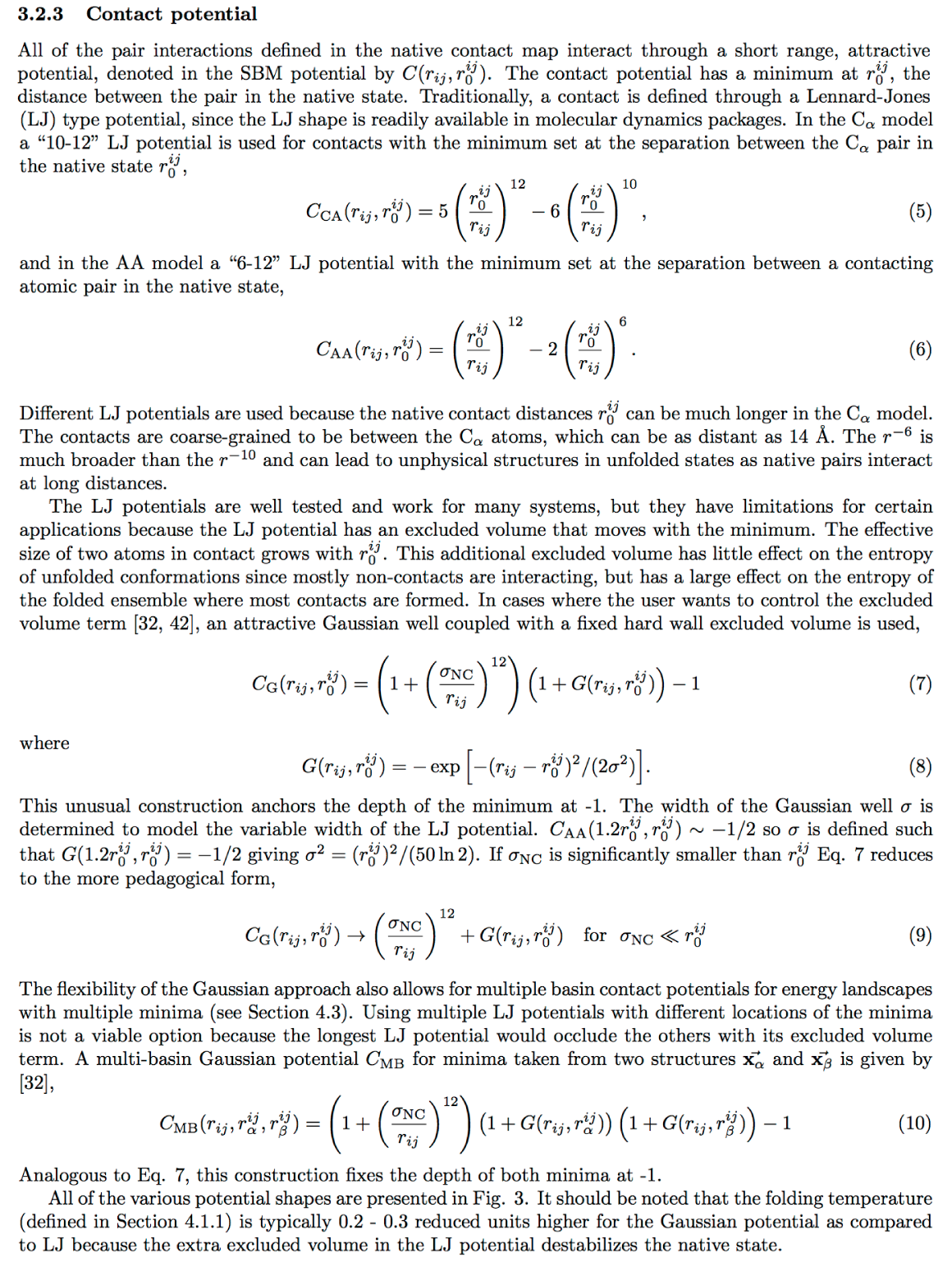# Gaussian contact potentials

## Bringing freedom of creativity to the SMOG modeller!

A tarball is available here. The extension is added to GROMACS v4.5.4. Compile GROMACS as you would any distribution, nothing special is required at compile time. This provides three new non-bonded pair interactions that can be included in the `[ pairs ]` section of the topology (.top). README.SBM in the root directory has helpful information that is reproduced below. A script that will convert a `[ pairs ]` section between Lennard-Jones and Gaussians in the all-atom model is available here. The width parameter (sigma) for Calpha models is typically a constant 0.5 Å, regardless of the native distance.

Here is an excerpt from a book chapter (Springer book) on the contact potentials in SMOG which will introduce the Gaussians.### Example topology

``` Excerpt of topology *************** [ pairs ] ;normal LJ 6-12 pairs 4 497 1 6.7105667E-02 1.4373939E-03 5 183 1 8.8991727E-02 1.6851818E-03 5 338 1 1.5051600E-02 1.2052582E-04 5 339 1 1.2527527E-02 8.3485455E-05 6 183 1 5.7529955E-02 7.0429500E-04 ; .... ;standalone Gaussian well 1 316 5 0.818992 0.279187 0.0474239 ; ... ;Gaussian well with connected a/r^12 repulsive core ;(must be used with [ exclusions ]) 1 316 6 0.818992 0.279187 0.0474239 0.59605E-09 ; ... ;dual basin Gaussian with connected a/r^12 repulsive core ;(must be used with [ exclusions ]) 1 316 7 0.819006 0.279187 0.0474239 0.426216 0.072399 0.59605E-09 ; ... ***************************************************** ```

### Single Gaussian contact potential``` bare Gaussian potential: V_ij = -A exp( - ( r - mu )^2 / ( 2 sigma^2 ) ) selected in the [ pairs ] section; ; i j ftype A mu sigma with ftype = 5 note that the amplitude of the Gaussian is -A. This can be used with a separate repulsion term or without it. ---------------------------------------------- combined Gaussian - r^-12 potential: V_ij = A({ 1 + (1/A)a/(r^12) }{ 1 - exp[ - ( r - mu )^2 / ( 2 sigma^2 ) ] }-1) = A({ 1 + R/A }{ 1 - G } - 1) = R - AG - RG this construction keeps the minimum of V fixed at ( mu, -A ) selected in the [ pairs ] section of the .top file ; i j ftype A mu sigma a with ftype = 6 note that the amplitude of the Gaussian is -A. IMPORTANT: the term R (and its derivative) now IS calculated here, the separate repulsion defined in [ atomtypes ] should be removed for each pair using this potential by [ exclusions ] : ; i j note that the section [ bonds ] must appear before [ exclusions ] (as of 3.3) `````` two Gaussians & r^-12 repulsion: V_ij = A[ (1+R/A) (1-F) (1-G) - 1] = R - AF - AG + AFG - FR - GR + FGR F = exp( - ( r - mu1 )^2 / ( 2 sigma1^2 ) ) G = exp( - ( r - mu2 )^2 / ( 2 sigma2^2 ) ) R = a/(r^12) selected in the [ pairs ] section; ; i j ftype A mu1 sigma1 mu2 sigma2 a with ftype = 7 both Gaussians get the common amplitude -A, minima of the resulting function are (mu1,-A) & (mu2,-A) as for the single Gaussian, [ exclusions ] are required ```